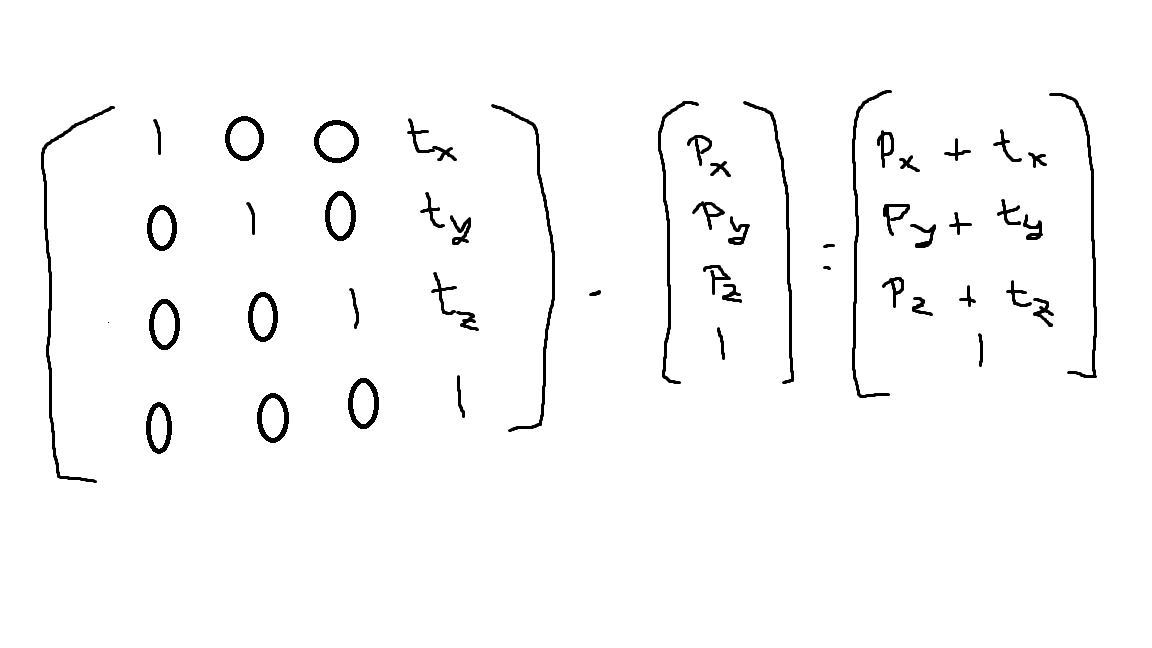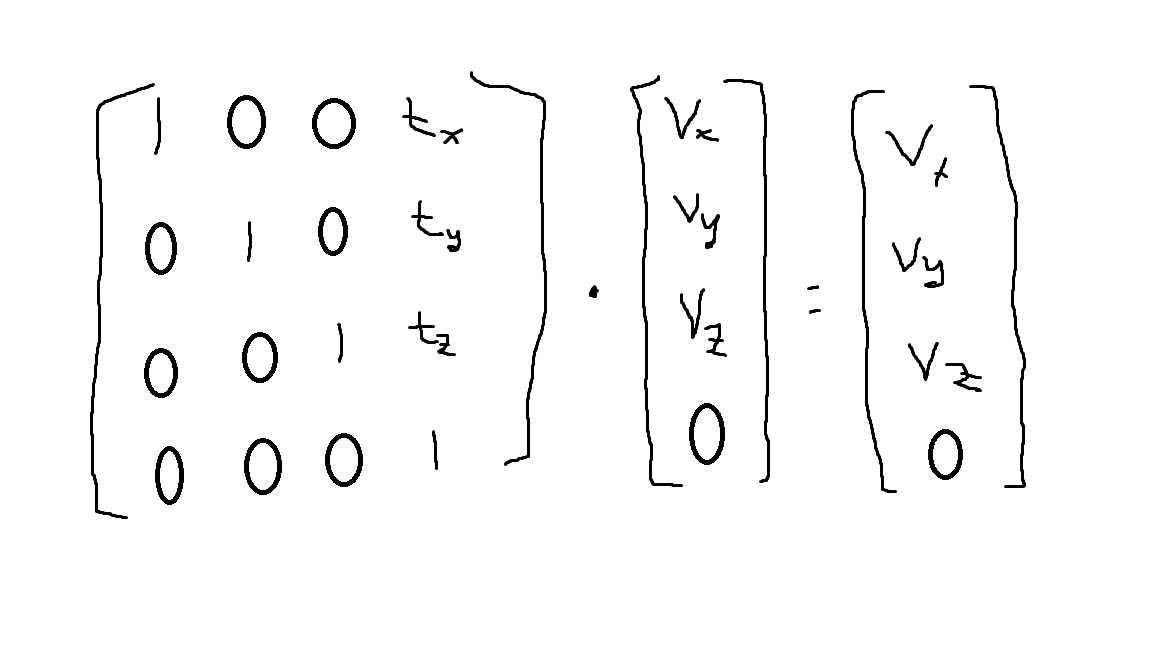How to multiply a QMatrix4x4 * Qvector4D and save the result in a QVector4D variable

• I want to multiply a QMatrix4x4 with a QVector4D and save it in a class variable but I can't do it.

void camera::pan(float angulo)
{
QMatrix4x4 translationMatrix1 = QMatrix4x4();
QMatrix4x4 rotationMatrix = QMatrix4x4();
QMatrix4x4 translationMatrix2 = QMatrix4x4();

translationMatrix1.translate( -this->position);
translationMatrix2.translate(this->position);
QMatrix4x4 transformacion = translationMatrix2 * rotationMatrix * translationMatrix1;

this->lookAt = transformacion * QVector4D(this->lookAt, 1);
this->u = transformacion * QVector4D(this->u, 0);
this->v = transformacion* QVector4D(this->v, 0);
this->n = transformacion * QVector4D(this->n, 0);
calcularMatrices();
}

lookAt, u, v and n are QVector3D variable.
Thank you.

• Hi @JesusM

The function rotate needs the angle in degrees, not in radians.

Depending on your system of coordinates, the fourth dimension wvalues may need to set to 1.

And the transform (translate, rotate, translate) could be also performed in only one matrix:

If lookAt, u, v and n are QVector3D, you don't need to convert them to a QVector4D: https://doc.qt.io/qt-5/qmatrix4x4.html#operator-2a-2

void camera::pan(float angulo)
{
QMatrix4x4 transform = QMatrix4x4();

transform.translate( -this->position);
transform.rotate(angulo, QVector3D(0, 1, 0));
transform.translate(this->position);

this->lookAt = transform * this->lookAt;
this->u = transform * this->u;
this->v = transform * this->v;
this->n = transform * this->n;

calcularMatrices();
}

• Hi @beecksche, thanks for helping.
Yoy are right, they are QVector3D, I was wrong.
According to the w coord, points must have w to 1 and vectors must have it to 0, but were do I put it? If i had the Vector3D i don't have the w coord and I only need it for the geometry operations but if I use the Vector3D I am not saying anywhere what is its w coord.

• @JesusM

Whats the difference between a Point and a Vector?

As far as I understand your function, you want to rotate 4 vectors lookAt, u, v and n in 3D space around the y-axis, right?

For the transformation there is no need for the fourth dimension w:

The QMatrix4x4 class represents a 4x4 transformation matrix in 3D space.

• @beecksche I will put you into context,
This operations are for a camera move, I have a point where I am looking (lookAt) and 3 vectors(u,v,n) that define my camera axis which are not the same as my world axis. If I want to rotate, I have to apply the same transformations to the point and the vectors, but they don't react the same way. I made an example in paint (sorry xD) to show you how translation, for example, affects in different ways to vectors and points and that's why it is important to have the w coord.

Translation Matrix applied to a point:Traslation Matrix applied to a vector:As you can see vectors are not translated and, instead of doind different transformation between vectors and points, you specify the w coord so when the operation is applied it will affect in different ways to both of them. That's why I need to have the w coord and I need to be able to change it.
The representation of a point and a vector with the w coord is called homogeneous coord and it is used in graphics applications (as mine) to make calculations.

• I was trying things and I found a solution, I will post it here if someone has the same problem:

void camera::pan(float angulo)
{
QMatrix4x4 translationMatrix1 = QMatrix4x4();
QMatrix4x4 rotationMatrix = QMatrix4x4();
QMatrix4x4 translationMatrix2 = QMatrix4x4();

translationMatrix1.translate( -this->position);
rotationMatrix.rotate(angulo, QVector3D(0, 1, 0));
translationMatrix2.translate(this->position);
QMatrix4x4 transformacion = translationMatrix2 * rotationMatrix * translationMatrix1;

QVector4D aux;
aux = transformacion * QVector4D(this->lookAt,1);
this->lookAt = aux.toVector3D();

aux = transformacion * QVector4D(this->u,0);
this->u = aux.toVector3D();

aux =  transformacion* QVector4D(this->v,0);
this->v = aux.toVector3D();

aux = transformacion * QVector4D(this->n,0);
this->n = aux.toVector3D();

calcularMatrices();
}

What I just did is to use an auxiliar vector which is a QVector4D to make the operations and then I use a method called toVector3D() to save the result (x,y,z) in my variable as I only need the w coord for the operations.

• @JesusM

The solutions should be fine, but homogenous coordinates are defined with:

float x, y, z; //euclidean coordinates
float w; // weight

float hx = x*w; // homogenous coordinates
float hy = y*w; // homogenous coordinates
float hz = z*w; // homogenous coordinates

x = hx / w; // back to euclidean
y = hy / w; // back to euclidean
z = hz / w; // back to euclidean

If w = 1the homogenous coordinates are equal to the euclidean.

In your case w = 0, so they cannot be homogenous coordinates. Also by calling toVector3D() the w coordinate will be dropped (toVector3DAffine() will convert homogenous to euclidean coordinates).

You just skip the translation (tx, ty and tz) by setting w=0. Actually you should use two different tranfomation matrices, to clarify what operations you want to do.

• @beecksche I need it to be dropped. The division by w is done in the rendering pipeline so I dont need to do it. I just need it to operate and then I dropped it.
The code I had first was

this->v = transformacion * glm::vec4(this->v, 0)

It worked because glm has the equal operator overcharged so I could make a glm::vec4, assignation to a glm::vec3 and glm just dropped out the w coord.
Now I am translating this code to Qt without using glm but Qt does not have the equal operator overcharge so I had to do it in two steps.
You are right with the Euclidean coord but I dont need to do it.